GMAT  >  Test: Inequalities + Absolute Value (Modulus)

# Test: Inequalities + Absolute Value (Modulus)

Test Description

## 15 Questions MCQ Test General Test Preparation for CUET | Test: Inequalities + Absolute Value (Modulus)

Test: Inequalities + Absolute Value (Modulus) for GMAT 2022 is part of General Test Preparation for CUET preparation. The Test: Inequalities + Absolute Value (Modulus) questions and answers have been prepared according to the GMAT exam syllabus.The Test: Inequalities + Absolute Value (Modulus) MCQs are made for GMAT 2022 Exam. Find important definitions, questions, notes, meanings, examples, exercises, MCQs and online tests for Test: Inequalities + Absolute Value (Modulus) below.
Solutions of Test: Inequalities + Absolute Value (Modulus) questions in English are available as part of our General Test Preparation for CUET for GMAT & Test: Inequalities + Absolute Value (Modulus) solutions in Hindi for General Test Preparation for CUET course. Download more important topics, notes, lectures and mock test series for GMAT Exam by signing up for free. Attempt Test: Inequalities + Absolute Value (Modulus) | 15 questions in 15 minutes | Mock test for GMAT preparation | Free important questions MCQ to study General Test Preparation for CUET for GMAT Exam | Download free PDF with solutions
 1 Crore+ students have signed up on EduRev. Have you?
Test: Inequalities + Absolute Value (Modulus) - Question 1

### If -1 < x < 0, which of the following must be true? I. x3 < x2 II. x5 < 1 – x III. x4 < x2

Detailed Solution for Test: Inequalities + Absolute Value (Modulus) - Question 1

There are two characteristics of x that dictate its exponential behavior.  First of all, it is a decimal with an absolute value of less than 1. Secondly, it is a negative number.

I.  True.  x3 will always be negative (negative × negative × negative = negative), and x2 will always be positive (negative × negative = positive), so x3 will always be less than x2.

II. True.  x5 will always be negative, and since x is negative, 1 – x will always be positive because the double negative will essentially turn 1 – x  into 1 + |x|.  Therefore, x5 will always be less than 1 – x.

III. True.  One useful method for evaluating this inequality is to plug in a number for x.  If x = - 0.5,

x4 = (-0.5)4 = 0.0625

x2 = (-0.5)2 = 0.25

To understand why this works, it helps to think of the negative aspect of x and the decimal aspect of x separately.

Because x is being taken to an even exponent in both instances, we can essentially ignore the negative aspect because we know the both results will be positive.

The rule with decimals between 0 and 1 is that the number gets smaller and smaller in absolute value as the exponent gets bigger and bigger.  Therefore, x4 must be smaller in absolute value than x2.

Test: Inequalities + Absolute Value (Modulus) - Question 2

### If a – b > a + b, where a and b are integers, which of the following must be true? I. a < 0 II. b < 0 III. ab < 0

Detailed Solution for Test: Inequalities + Absolute Value (Modulus) - Question 2

We are given the inequality a – b > a + b. If we subtract a from both sides, we are left with the inequality -b > b. If we add b to both sides, we get 0 > 2b. If we divide both sides by 2, we can rephrase the given information as 0 > b, or b is negative.

I. FALSE: All we know from the given inequality is that 0 > b. The value of a could be either positive or negative.

II. TRUE: We know from the given inequality that 0 > b. Therefore, b must be negative.

III. FALSE: We know from the given inequality that 0 > b. Therefore, b must be negative. However, the value of a could be either positive or negative. Therefore, ab could be positive or negative.

Test: Inequalities + Absolute Value (Modulus) - Question 3

### If |a| = 1/3 and |b| = 2/3, which of the following CANNOT be the result of a + b?

Detailed Solution for Test: Inequalities + Absolute Value (Modulus) - Question 3

Given that |a| = 1/3, the value of a could be either 1/3 or -1/3.  Likewise, b could be either 2/3 or -2/3. Therefore, four possible solutions to a + b exist, as shown in the following table: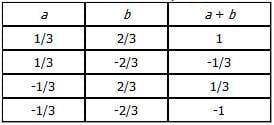2/3 is the only answer choice that does not represent a possible sum of a + b.

Test: Inequalities + Absolute Value (Modulus) - Question 4

If |a| = |b|, which of the following must be true?
I. a = b
II. |a| = -b
III. -a = -b

Detailed Solution for Test: Inequalities + Absolute Value (Modulus) - Question 4

Because we know that |a| = |b|, we know that a and b are equidistant from zero on the number line. But we do not know anything about the signs of a and b (that is, whether they are positive or negative). Because the question asks us which statement(s) MUST be true, we can eliminate any statement that is not always true. To prove that a statement is not always true, we need to find values for a and b for which the statement is false.
I. NOT ALWAYS TRUE: a does not necessarily have to equal b. For example, if a = -3 and b = 3, then |-3| = |3| but -3 ≠ 3.
II. NOT ALWAYS TRUE: |a| does not necessarily have to equal -b. For example, if a = 3 and b = 3, then |3| = |3| but |3| ≠ -3.
III. NOT ALWAYS TRUE: -a does not necessarily have to equal -b. For example, if a = -3 and b = 3, then |-3| = |3| but -(-3) ≠ -3.

Test: Inequalities + Absolute Value (Modulus) - Question 5

Which of the following inequalities has a solution set that when graphed on the number line, is a single
segment of finite length?

Detailed Solution for Test: Inequalities + Absolute Value (Modulus) - Question 5

A. x4>=1=>(x4-1)>=0=>(x2-1)(x2+1)>=0=>(x+ 1)(x-1) (x2+1)>=0
(x2+1)>0, so (x+1)(x-1)>=0 =>x<-1,x>1
B. x2<=27=>(x3-33)<=0=>(x-3)(X2+3x+32)<=0, with d=b2-4ac we can know that (X2+3x+32) has no solution, but we know that (X2+3x+32)=[(x+3/2)2+27/4]>0, then, (x-3)<=0, x<=3
C. x2>=16, [x2-42]>=0, (x-4)(x+4)>=0, x>4,x<-4
D. 2<=IxI<=5, 2<=IxI, x>=2, or x<=2

Test: Inequalities + Absolute Value (Modulus) - Question 6

If |x – (9/2)| = 5/2, and if y is the median of a set of p consecutive integers, where p is odd, which of the
following must be true?
I. xyp is odd
II. xy(p2 + p) is even
III. x2y2p2 is even

Detailed Solution for Test: Inequalities + Absolute Value (Modulus) - Question 6

The rules of odds and evens tell us that the product will be odd if all the factors are odd, and the product will be even if at least one of the factors is even.  In order to analyze the given statements I, II, and III, we must determine whether x and y are odd or even.  First, solve the absolute value equation for x by considering both the positive and negative values of the absolute value expression.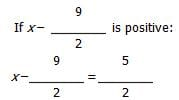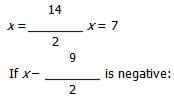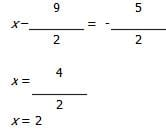Therefore, x can be either odd or even.

Next, consider the median (y) of a set of p consecutive integers, where p is odd.  Will this median necessarily be odd or even?  Let's choose two examples to find out:

Example Set 1:  1, 2, 3  (the median y = 2, so y is even)

Example Set 2:  3, 4, 5, 6, 7 (the median y = 5, so y is odd)

Therefore, y can be either odd or even.

Now, analyze the given statements:

I.  UNCERTAIN:  Statement I will be true if and only if x, y, and p are all odd.  We know p is odd, but since x and y can be either odd or even we cannot definitively say that xyp will be odd.  For example, if x = 2 then xyp will be even.

II.  TRUE:  Statement II will be true if any one of the factors is even.  After factoring out a p, the expression can be written as xyp(p + 1).  Since p is odd, we know (p + 1) must be even.  Therefore, the product of xyp(p + 1) must be even.

III.  UNCERTAIN:  Statement III will be true if any one of the factors is even.  The expression can be written as xxyypp.  We know that p is odd, and we also know that both x and y could be odd.

Test: Inequalities + Absolute Value (Modulus) - Question 7

If a, b, c, and d are integers and ab2c3d4 > 0, which of the following must be positive?
I. a2cd
II. bc4d
III. a3c3d2

Detailed Solution for Test: Inequalities + Absolute Value (Modulus) - Question 7

First, let’s try to make some inferences from the fact that ab2c3d4 > 0. Since none of the integers is equal to zero (their product does not equal zero), b and d raised to even exponents must be positive, i.e. b2 > 0 and d4 > 0, implying that b2d4 > 0. If b2d4 > 0 and ab2c3d4 > 0, the product of the remaining variables, a and c3 must be positive, i.e. ac3 > 0. As a result, while we do not know the specific signs of any variable, we know that ac > 0 (because the odd exponent c3 will always have the same sign as c) and therefore a and c must have the same sign—either both positive or both negative.

Next, let’s evaluate each of the statements:

I. UNCERTAIN: While we know that the even exponent a2 must be positive, we do not know anything about the signs of the two remaining variables, c and d. If c and d have the same signs, then cd > 0 and a2cd > 0, but if c and d have different signs, then cd < 0 and a2cd < 0.

II. UNCERTAIN: While we know that the even exponent c4 must be positive, we do not know anything about the signs of the two remaining variables, b and d. If b and d have the same signs, then bd > 0 and bc4d > 0, but if b and d have different signs, then bd < 0 and bc4d < 0.

III. TRUE: Since a3c3 = (ac)3 and a and c have the same signs, it must be true that ac > 0 and   (ac)3 > 0. Also, the even exponent d2 will be positive. As a result, it must be true that a3c3d2 > 0.

Test: Inequalities + Absolute Value (Modulus) - Question 8

If 3|3 – x| = 7, what is the product of all the possible values of x?

Detailed Solution for Test: Inequalities + Absolute Value (Modulus) - Question 8

When solving an absolute value equation, it helps to first isolate the absolute value expression:

3|3 – x| = 7
|3 – x| = 7/3

When removing the absolute value bars, we need to keep in mind that the expression inside the absolute value bars (3 – x) could be positive or negative.  Let's consider both possibilities:

When (3 – x) is positive:
(3 – x) = 7/3
3 – 7/3 = x
9/3 – 7/3 = x
x = 2/3

When (3 – x) is negative:
-(3 – x) = 7/3
x – 3 = 7/3
x = 7/3 + 3
x = 7/3 + 9/3
x = 16/3

So, the two possible values for x are 2/3 and 16/3.  The product of these values is 32/9.

Test: Inequalities + Absolute Value (Modulus) - Question 9

If √[(x + 4)2] = 3,which of the following could be the value of x – 4?

Detailed Solution for Test: Inequalities + Absolute Value (Modulus) - Question 9

Square both sides of the given equation to eliminate the square root sign:
(x + 4)2 = 9

Remember that even exponents “hide the sign” of the base, so there are two solutions to the equation: (x + 4) = 3 or (x + 4) = -3. On the GMAT, the negative solution is often the correct one, so evaluate that one first.
(x + 4) = -3
x = -3 – 4
x = -7

Watch out! Although -7 is an answer choice, it is not correct. The question does not ask for the value of x, but rather for the value of x – 4 = -7 – 4 = -11.
Alternatively, the expression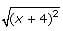can be simplified to |x + 4|, and the original equation can be solved accordingly.
If |x + 4| = 3, either x = -1 or x = -7

Test: Inequalities + Absolute Value (Modulus) - Question 10

If |x | + |y | = -x – y and xy does not equal 0, which of the following must be true?

Detailed Solution for Test: Inequalities + Absolute Value (Modulus) - Question 10

|x| + |y| = -x - y
|x| + |y| = -(x+y)

I know that |x| + |y| is always (+). But the other side is -(x+y).
To make it positive, we multiple (-) with (-).

Thus, (x+y) is (-) or x+y < 0

Test: Inequalities + Absolute Value (Modulus) - Question 11

If x > 0, what is the least possible value for x + (1/x)?

Detailed Solution for Test: Inequalities + Absolute Value (Modulus) - Question 11

When we plug a few values for x, we see that the expression doesn't seem to go below the value of 2.  It is important to try both fractions (less than 1) and integers greater than 1. Let's try to mathematically prove that this expression is always greater than or equal to 2. Is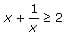Since x > 0, we can multiply both sides of the inequality by x: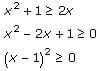The left side of this inequality is always positive, so in fact the original inequality holds.

Test: Inequalities + Absolute Value (Modulus) - Question 12

If (a – b)c < 0, which of the following cannot be true?

Detailed Solution for Test: Inequalities + Absolute Value (Modulus) - Question 12

If (a – b)c < 0, the expression (a – b) and the variable c must have opposite signs.
(A) UNCERTAIN:  If a < b, a – b would be negative.  It is possible for a – b to be negative according to the question.

(B) UNCERTAIN:  It is possible for c to be negative according to the question.

(C) UNCERTAIN:  This means that -1 < c < 1, which is possible according to the question.

(D) FALSE:  If we rewrite this expression, we get  ac – bc > 0.  Then, if we factor this, we get: (a – b)c > 0. This directly contradicts the information given in the question, which states that (a – b)c < 0.

(E) UNCERTAIN:  If we factor this expression, we get (a + b)(a – b) < 0.  This tells us that the expressions a + b and a – b have opposite signs, which is possible according to the question.

Test: Inequalities + Absolute Value (Modulus) - Question 13

If |ab| > ab, which of the following must be true?
I. a < 0
II. b < 0
III. ab < 0

Detailed Solution for Test: Inequalities + Absolute Value (Modulus) - Question 13

If  |ab| > ab, ab must be negative.  If ab were positive the absolute value of ab would equal ab. We can rephrase this question: "Is ab < 0?"

I.  UNCERTAIN:  We know nothing about the sign of b.

II.  UNCERTAIN:  We know nothing abou the sign of a.

III.  TRUE:  This answers the question directly.

Test: Inequalities + Absolute Value (Modulus) - Question 14

If b < c < d and c > 0, which of the following cannot be true if b, c and d are integers?

Detailed Solution for Test: Inequalities + Absolute Value (Modulus) - Question 14

Since c > 0 and d > c, c and d must be positive. b could be negative or positive. Let's look at each answer choice:
(A) UNCERTAIN:  bcd could be greater than zero if b is positive.

(B) UNCERTAIN:  b + cd could be less than zero if b is negative and its absolute value is greater than that of cd.  For example: b = -12, c = 2, d = 5 yields -12 + (2)(5) = -2.

(C) FALSE:  Contrary to this expression, b – cd must be negative. We could think of this expression as b + (-cd).  cd itself will always be positive, so we are adding a negative number to b. If b < 0, the result is negative.  If b > 0, the result is still negative because a positive b must still be less than cd (remember that b < c < d and b, c and d are integers).

(D) UNCERTAIN:  This is possible if b is negative.

(E) UNCERTAIN:  This is possible if b is negative.

Test: Inequalities + Absolute Value (Modulus) - Question 15

If ab > cd and a, b, c and d are all greater than zero, which of the following CANNOT be true?

Detailed Solution for Test: Inequalities + Absolute Value (Modulus) - Question 15

Let's look at the answer choices one by one:
(A) POSSIBLE: c can be greater than b if a is much bigger than d.  For example, if c = 2, b = 1, a = 10 and d = 3, ab (10) is still greater than cd (6), despite the fact that c > b.
(B) POSSIBLE: The same reasoning from (A) applies.
(C) IMPOSSIBLE:  Since a, b, c and d are all positive we can cross multiply this fraction to yield ab < cd, the opposite of the inequality in the question.
(D) DEFINITE:  Since a, b, c and d are all positive, we can cross multiply this fraction to yield ab > cd, which is the same inequality as that in the question.
(E) DEFINITE: Since a, b, c and d are all positive, we can simply unsquare both sides of the inequality. We will then have cd < ab, which is the same inequality as that in the question.

## General Test Preparation for CUET

90 videos|107 docs|356 tests
 Use Code STAYHOME200 and get INR 200 additional OFF Use Coupon Code
Information about Test: Inequalities + Absolute Value (Modulus) Page
In this test you can find the Exam questions for Test: Inequalities + Absolute Value (Modulus) solved & explained in the simplest way possible. Besides giving Questions and answers for Test: Inequalities + Absolute Value (Modulus), EduRev gives you an ample number of Online tests for practice

## General Test Preparation for CUET

90 videos|107 docs|356 tests